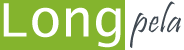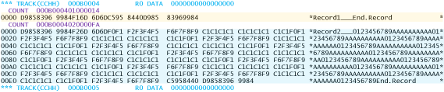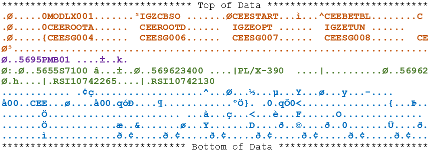[Image_Link]
 longpelaexpertise.com.au/ezine/RECFMU.php?ezinemode=printfriend# technical: RECFM=U: What Exactly Is It?

When I first started as a systems programmer in 1989, I was told that load libraries were defined with RECFM=U: "undefined" record format. But what exactly does "undefined" mean. And is there any reason to use RECFM=U for anything other than load libraries?

## What It Isn't

Before we talk about what RECFM=U is, let's talk about what it isn't.

Normally, datasets are defined as having fixed or variable-length records: RECFM=F, or RECFM=V. Fixed length records are exactly that: fixed. If we define a dataset with LRECL=80, every record is 80 bytes, whether we need it or not.

This can waste space when we have records of different lengths. For this, RECFM=V is the go. Every record starts with a record-descriptor word (RDW) holding (amongst other things), the record length. This is followed by the record contents.

Now, programs (and programmers) think in terms of records. But disks think (well, more accurately, they work) in terms of blocks. Every I/O to disk is one block. With RECFM=F and RECFM=V, there is one record per block. If we read or write five records, we need to do five I/Os (EXCPs). This is slow.

To make things faster, we almost always used 'blocked' datasets. This means that there can be more than one record in each block. So, with a large enough blocksize, we could read or write our five records in one EXCP. Some access methods like QSAM enhance this by including several blocks into one EXCP: chained scheduling. But that's another story.

We usually want to specify the largest blocksize we can, to fit as many records as possible into one block. The largest blocksize possible is 32k, but all contents of a block must say on the same track. Most disk devices pretend to be 3390s: track size of 56,664 bytes. If we specify a 32k blocksize, we'll only use 32k of the track, and waste the rest.

Best practices tell us to use blocks that are half a track in size (half-track blocks). We can fit two blocks on a track with minimal wastage, while maximizing the number of records that can fit in a block. Blocked datasets are defined with RECFM=FB and RECFM=VB for fixed and variable length datasets respectively. So let's see what a FB block looks like. We've defined a RECFM=FB dataset with LRECL=256, BLKSIZE=27904 (half-track blocking). We have two records: one of length 20, one of length 250.

One of the brilliant features of DFSMSdss (DSS) is that it can print out the contents of a disk location. Here's a sample job to print out the disk contents for our dataset:

```//STEP1      EXEC PGM=ADRDSSU
//SYSPRINT   DD   SYSOUT=*
//VOL1       DD   DIS=SHR,VOL=SER=VOL001
//SYSIN      DD   *
PRINT DATASET(MY.DATASET) IDD(VOL1) ALLDATA```

Here's the output for our dataset:We can see our two records (one shaded in orange, one in blue). Above these is a line in purple (COUNT) showing the beginning of our block:

`COUNT  004E000101000200`

Let's look at this COUNT value from left to right:

• X'004E': cylinder number where the block resides (often written as CC)
• X'0001': track number on this cylinder where the block resides (often written as HH for head number). This block is on the first track of the cylinder
• x'01': the block number on the track. This is the first block on the track
• x'000200': the length of our block (512 bytes).

But wait. Our block is 512 bytes long, but we've defined the blocksize of our dataset as 27904. So what's going on? We only have two records in our dataset. So, the block is 512 bytes, holding both our records. The remaining 27392 bytes of our block are reserved, and used when we add more records.

We can see that the first record, although only 20 bytes long, is from offset x'0000' to x'00FF' of our block: it uses up 256 bytes: our fixed record length. We can see our second record start at offset x'0100', and finish at x'01FF'. So our two records are in the same block.

## What RECFM=U Is

RECFM=U is a little different. First: only one record per block (just like RECFM=F and RECFM=V). If we define a RECFM=U dataset with a blocksize of 32k, then you'd think we could only store records of length 32k. But that's not true: RECFM=U records are variable length. How can this be?

Suppose we have a sequential RECFM=U dataset with a blocksize of 32760 bytes. Every record written to this dataset must be 32760 bytes or less in length. But if the record we are writing is only 1000 bytes, then it written to disk as a 1000-byte block (a 'short' block). So, we could almost think of RECFM=U as 'variable length blocks.'

## RECFM=U Blocks

Let's prove this. We created a sequential RECFM=U dataset with BLKSIZE=32760. We wrote the same two records as above: one 25 bytes long, one 250 bytes long. We used DFDSS to print the dataset. Here's the output:We can see two COUNT lines in purple: two blocks. The first record is in the first block, of length x'14' (20 bytes). The other is in a different block of length x'FA': 250 bytes.

## Working with RECFM=U

RECFM=U datasets can be browsed using normal ISPF screens. But what if we want to update a record in a RECFM=U dataset? Try to edit a RECFM=U dataset in ISPF, and you'll see that it doesn't work. You can write a program to read, then update a record. Some utilities like IBM Filemanager can also help. However, with RECFM=U, updated records cannot change length. You can update the contents only. If you update a record with a longer record, it is truncated. If it's shorter, it is padded.

So RECFM=U are really good for data that is written once: like program objects.

Most languages support RECFM=U, including IBM Enterprise COBOL, Enterprise PL/I and IBM XL C/C++. From z/OS 2.1, REXX also supports RECFM=U. However, some utilities don't work with RECFM=U. For example, DFSORT cannot output to a RECFM=U dataset.

If our records are variable length, and our blocks are the same size as our records, then what should we use as a record length (LRECL) when allocating a RECFM=U dataset? Doesn't matter; LRECL is ignored for RECFM=U datasets. Most people just specify RECFM=0.

## Uses for RECFM=U

RECFM=U dataset are useful when we're dealing with variable length data that we want to process as is: such as binary data. Unlike RECFM=V, we don't have to worry about record descriptor words: we can simply read the data as is. This makes them ideal for load modules and project objects. Take a look at an ISPF browse screen for a COBOL program:There are several records doing different things. Some hold information about external references (in brown), others have details about the binder version, and when it was bound (purple). And of course, there are the records with the actual module text (blue). You can see that these records are of differing lengths, and remember that each is a different block. Program objects are rarely updated (we could use AMASPZAP, but this isn't done often). Rather, we simply recreate the module every time it is bound. So RECFM=U is perfect for load modules and program objects.

But other uses of RECFM=U datasets aren't common. Today, there are better options around (such as VSAM linear datasets).

## Opening Files with RECFM=U

One interesting feature of RECFM=U is that is can let us view a dataset 'in the raw'. Consider the following JCL that executes a REXX in batch:

```//STEP1  EXEC  PGM=IKJEFT1B
//SYSTSPRT DD  SYSOUT=*
//SYSEXEC  DD  DISP=SHR,DSN=USER1.CNTL
//DD1      DD  DISP=OLD,DSN=USER1.TEST.RECFMVB,RECFM=U,DSORG=PS,
//         BLKSIZE=32760
//SYSTSIN  DD  *
%TEST2```

USER1.TEST.RECFMVB is an existing sequential dataset with LRECL=256, RECFM=VB, BLKSIZE=27998. But in this job, we're specifying RECFM=U, and a different blocksize. We're opening this dataset as a RECFM=U dataset.

Here's the REXX TEST2:

```/* Rexx */
i=0
"Execio * DISKR DD1 (Finis Stem inrec."
Do i = 1 to inrec.0
reclen = LENGTH(inrec.i)
Say "Record" i", Length="reclen
Say inrec.i
End
Exit 0```

Nothing special: just reads every record, and displays the result. Here's what we see (with a hexadecimal display below the contents of the record):

```Record 1, Length=69
First Line    A much longer second line ---------------------
04000000C89AA4D8980300C49A8849998894A88998498984666666666666666666666
05000E006992303955030010443803657590253654039550000000000000000000000```

Looking at our data from the left (in hex), we have:

• x'00450000' - this is the block descriptor word (BDW) that starts VB datasets
• x'000E0000' - the record descriptor word (RDW) that precedes a record. The first two bytes are the record length, including the 4 bytes of this RDW (x'000E' = 14 bytes)
• First Line - the contents of the first record ("First Line")
• X'00330000' - the next RDW (length x'0033' = 51 bytes)
• ... and so on

So we've read the 'raw' RECFM=VB file, seeing the descriptor words we normally don't see when we read a RECFM=VB file.

Using RECFM=U in this way can be powerful. You could scan a full PDS without opening every member (much faster). You could FTP a file to a z/OS RECFM=U dataset if you're not sure of the format (PDS or sequential, VB or FB, LRECL=?).

## Conclusion

RECFM=U is a way of storing variable length record without needing any record descriptor words: great for data where you don't want to strip out anything before using it. No blocking benefits built in: you need to do this yourself.

Today RECFM=U datasets are used for load modules and program objects, and not much else. RECFM=U could also be used for other applications needing to store variable length binary data in a way that can be accessed using QSAM or BPAM quickly, and is rarely updated once created.

Anyone writing a program to access non-VSAM data will use RECFM=FB or RECFM=VB rather than RECFM=U: easier, with better programming language support. The access method handles blocking for better performance, and a lot of the hard work is done for us.

David Stephens